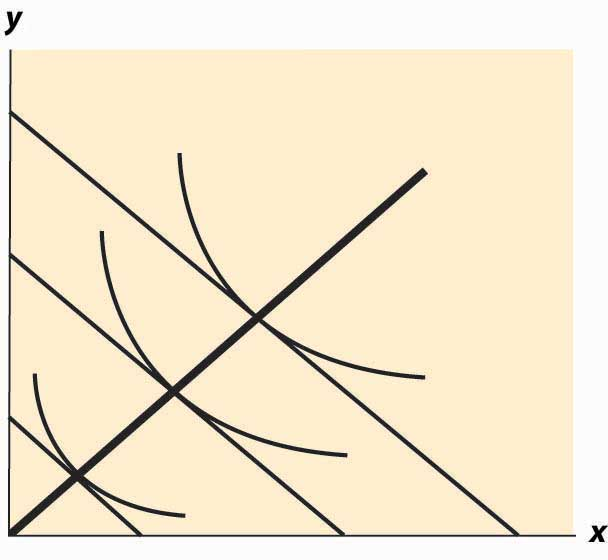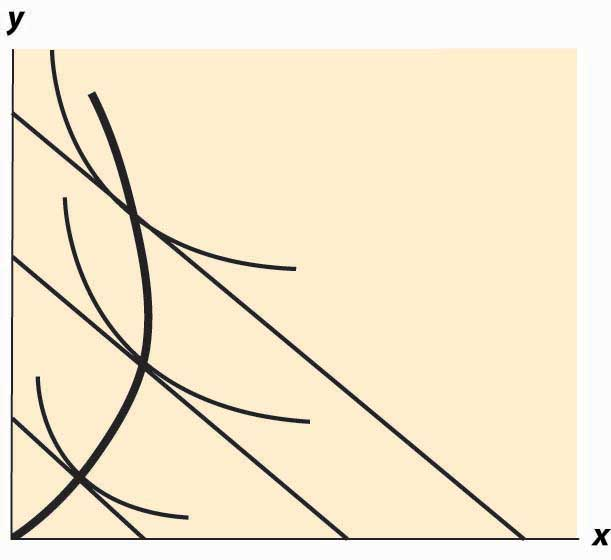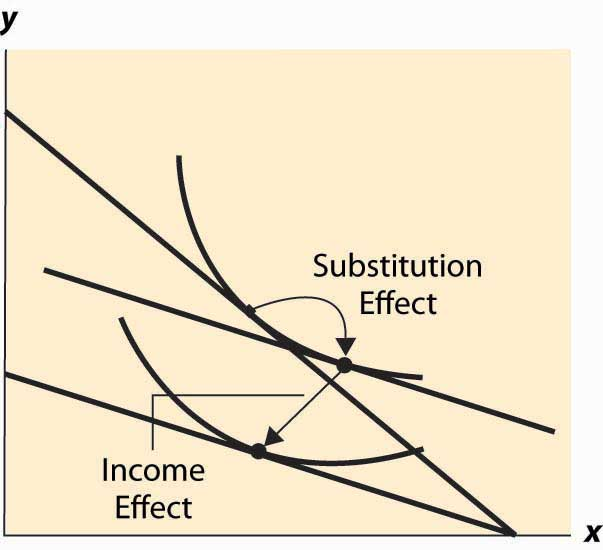## 12.6 Income Effects

### Learning Objective

1. How do consumers change their purchases when their income rises or falls?

Wealthy people buy more caviar than poor people. Wealthier people buy more land, medical services, cars, telephones, and computers than poorer people because they have more money to spend on goods and services and, overall, buy more of them. But wealthier people also buy fewer of some goods, too. Rich people buy fewer cigarettes and processed cheese foods. You don’t see billionaires waiting in line at McDonald’s, and that probably isn’t because they have an assistant to wait in line for them. For most goods, at a sufficiently high income, the purchase tends to trail off as income rises.

When an increase in income causes a consumer to buy more of a good, that good is called a normal good for that consumer. When the consumer buys less, the good is called an inferior good, which is an example of sensible jargon that is rare in any discipline. That is, an inferior good is any good whose quantity demanded falls as income rises. At a sufficiently low income, almost all goods are normal goods, while at a sufficiently high income, most goods become inferior. Even a Ferrari is an inferior good against some alternatives, such as Lear jets.

The curve that shows the path of consumption as income changes, holding prices constant, is known as an Engel curveGraph that shows the path of consumption as income changes, holding prices constant..The Engel curve is named for Ernst Engel (1821–1896), a statistician—not for Friedrich Engels, who wrote with Karl Marx. An Engel curve graphs (x(M), y(M)) as M varies, where x(M) is the amount of X chosen with income M, and similarly y(M) is the amount of Y. An example of an Engel curve is illustrated in Figure 12.11 "Engel curve".

Figure 12.11 Engel curveExample (Cobb-Douglas): Since the equations $x= αM p X , y= (1−α)M p Y$ define the optimal consumption, the Engel curve is a straight line through the origin with slope $(1−α) p X α p Y .$

An inferior good will see the quantity fall as income rises. Note that, with two goods, at least one is a normal good—they can’t both be inferior goods because otherwise, when income rises, less of both would be purchased. An example of an inferior good is illustrated in Figure 12.12 "Backward bending—inferior good". Here, as income rises, the consumption of x rises, reaches a maximum, and then begins to decline. In the declining portion, X is an inferior good.

The definition of the substitution effect now permits us to decompose the effect of a price change into a substitution effect and an income effect. This is illustrated in Figure 12.13 "Income and substitution effects".

What is the mathematical form of the income effect? This is actually more straightforward to compute than the substitution effect computed above. As with the substitution effect, we differentiate the conditions $M= p X x+ p Y y$ and $p X u 2 = p Y u 1 ,$ holding pX and pY constant, to obtain $dM= p X dx+ p Y dy$ and $p X ( u 12 dx+ u 22 dy)= p Y ( u 11 dx+ u 12 dy).$

Figure 12.12 Backward bending—inferior goodFigure 12.13 Income and substitution effectsThe second condition can also be written as $dy dx = p Y u 11 − p X u 12 p X u 22 − p Y u 12 .$

This equation alone defines the slope of the Engel curve without determining how large a change arises from a given change in M. The two conditions together can be solved for the effects of M on X and Y. The Engel curve is given by

$dx dM = p Y 2 u 11 −2 p X u 12 + p X 2 u 22 p X u 22 − p Y u 12$

and

$dy dM = p Y 2 u 11 −2 p X u 12 + p X 2 u 22 p Y u 11 − p X u 12 .$

Note (from the second-order condition) that good Y is inferior if $p Y u 11 − p X u 12 >0,$ or if $u 11 u 1 − u 12 u 2 >0,$ or $u 1 u 2$ is increasing in x. Since $u 1 u 2$ is locally constant when M increases, equaling the price ratio, and an increase in y increases $u 1 u 2$ (thanks to the second-order condition), the only way to keep $u 1 u 2$ equal to the price ratio is for x to fall. This property characterizes an inferior good—an increase in the quantity of the good increases the marginal rate of substitution of that good for another good.

### Key Takeaways

• When an increase in income causes a consumer to buy more of a good, that good is called a normal good for that consumer. When the consumer buys less, the good is called an inferior good. At a sufficiently high income, most goods become inferior.
• The curve that shows the path of consumption as income rises is known as an Engel curve.
• For the Cobb-Douglas utility, Engel curves are straight lines through the origin.
• Not all goods can be inferior.
• The effect of a price increase decomposes into two effects: a decrease in real income and a substitution effect from the change in the price ratio. For normal goods, a price increase decreases quantity. For inferior goods, a price increase decreases quantity only if the substitution effect is larger than the income effect.

### Exercises

1. Show that, in the case of perfect complements, the Engel curve does not depend on prices.
2. Compute the substitution effect and income effect associated with a multiplicative price increase Δ in pY—that is, multiplying pY by Δ > 1 for the case of the Cobb-Douglas utility $u( x,y )= x α y 1−α .$